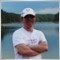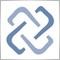# Persistence quality issue in standard library299

I have raised the quicksearch problem in CList and build 350 has fixed it.  However, why you didnt also check the quicksearch in all the array libraries?  Quicksearch they also use the old algo in CList and fail!

CList::QuickSearch

```   i=0;
j=m_data_total;
while(j>=i)
{
//--- ">>1" is quick division by 2
m=(j+i)>>1;
if(m<0 || m>=m_data_total) break;
t_node=GetNodeAtIndex(m);
if(t_node.Compare(element,m_sort_mode)==0) break;
if(t_node.Compare(element,m_sort_mode)>0)  j=m-1;
else                                       i=m+1;
}```

CArrayString::QuickSearch

```   i=0;
j=m_data_total-1;
while(j>=i)
{
//--- ">>1" is quick division by 2
m=(j+i)>>1;
if(m==0 || m==m_data_total-1) break;
t_string=m_data[m];
if(t_string==element) break;
if(t_string>element) j=m-1;
else                 i=m+1;
}```16963

Sorry. Fixed for all Arrays. Wait for next build, please.299

I re-tested CList again with 1 entry, it does not work.

void OnStart() {
CList list;
CString str1;
str1.Assign("a");
list.Sort(0);
CString str2;
str2.Assign("b");
CString *found=list.Search(GetPointer(str2));
if (found)
Print(found.Str());
}

"a" is printed and the logic searched for "b".299

by the way, if the same logic is copied to Arrays, it crashed because QuickSearch returns 1 where there is only 1 element in the array (index 0).299

```bool CArrayObj::Delete(int index)
{
//--- checking
if(index>=m_data_total) return(false);
//--- delete
if(index<0 || index<m_data_total-1) MemMove(index,index+1,m_data_total-index-1);
m_data_total--;
//---
return(true);
}```

I thought Delete method should delete the object at index, but instead it just over write the pointer without releasing it!  Took me 3 days to debug my code why a pointer is not released properly!!!299

Is anyone going to respond to fix this?

Memmove is never called if I want to delete the last element of the array, in this case index would be equal to m_data_total-1.  The pointer will not be released.6516

All changes will available at the next build. At this moment you can use attached file.

Files:
ArrayObj.mqh 32 kb16963299

Alexvd,

Thanks for your quick fix offer:

```if(index<m_data_total-1)
{
if(index>=0) MemMove(index,index+1,m_data_total-index-1);
}
else
{
if(CheckPointer(m_data[index])==POINTER_DYNAMIC) delete m_data[index];
}```

but i think you need to check the freemode flag before deleting.

Rosh,

I m disappointed that Build 353 does not fix this issue.16963

williamwong:

Rosh,

I m disappointed that Build 353 does not fix this issue.

I don't know what you say about. What issue do you exactly mean?299

Rosh:
I don't know what you say about. What issue do you exactly mean?
```bool CArrayObj::Delete(int index)
{
//--- checking
if(index>=m_data_total) return(false);
//--- delete
if(index<0 || index<m_data_total-1) MemMove(index,index+1,m_data_total-index-1);
m_data_total--;
//---
return(true);
}
```

Object is not deleted when index=m_data_total-1.